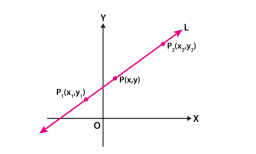# Geometry: 78014

Good day,
Even though it is a trivial task, I don’t know how to deal with it. This is analytic geometry:
Find all integers a, b, and c such that the line given by the equation ax+by=c passes through the points [4,3] and [−2,1].

a =  -12
b =  36
c =  60

### Step-by-step explanation:Did you find an error or inaccuracy? Feel free to write us. Thank you!

Tips for related online calculators
The line slope calculator is helpful for basic calculations in analytic geometry. The coordinates of two points in the plane calculate slope, normal and parametric line equation(s), slope, directional angle, direction vector, the length of the segment, intersections of the coordinate axes, etc.
Do you have a linear equation or system of equations and looking for its solution? Or do you have a quadratic equation?